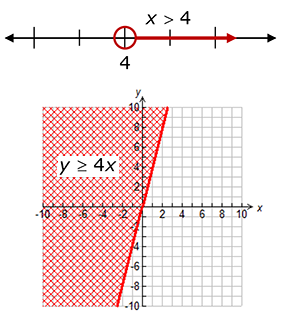Inequality

My Definition

Key Characteristics

The following are true of an inequality:

• It is a relationship between two expressions that may or may not be equal.

• It uses one of the following symbols:
> (greater than),
< (less than),
≥ (greater than or equal),
≤ (less than or equal),
≠ (not equal).

• It may have more than one solution or no solution.

ExampleNon-example

This process is called solving an equation. In this case, the process results in one value for x that makes the equation true.

-4(x -1) = 6x + 5 + 2x
-4x + 4 = 8x - 5
-12x = -9
x =
-9-12

x =
34

TEKS: 6(9)(A), 6(9)(B), 6(9)(C), 6(10)(A), 6(10)(B), 7(10)(A), 7(10)(B), 7(10)(C),
7(11)(A), 7(11)(B), 8(8)(A), 8(8)(B)